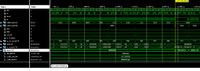[SOLVED]Interfacing UART with FPGA xilinx virtex 5, problem ?

Status
Not open for further replies.

Taki_comp

Member level 1I am trying to design a simple loop of communication system between pc and FPGA virtex 5, for this purpose I interfaced a BRAM with uart module, I am using VHDL as the hardware description language, the memory used is a 16 byte simple dual port BRAM ram with a width of 8-bits; is supposed to read 16 bytes of data from a terminal software and then sending them back to it, the problem is that I cannot write the first address(addra = 0) and the writing starts from the second address thus I can write only 15 bytes in one pass, here is the code of the fsm used to implement the system
Code:
proc_next_state: process(clk, reset, state)
begin
if reset = '1' then
state <= idle;
elsif (rising_edge(clk)) then
case state is
when idle =>
wea(0) <= '0' ;
dina <= rx_byte; -- input of BRAM's port A.
ENB <= '0'; -- Enable signal for port B
tx_DV <= '0'; -- data valid signal for uart transmitting interface.
tx_byte <=(others => '0'); -- byte to be loaded to uart transmitting interface
if rx_dv = '1' then -- data valid signal for uart receiving interface
state <= writing; -- if rx_dv is asserted move to the writing state
else
state <= idle;                              -- keep idle
end if;

when writing =>
if addra = "1111" then -- if the whole block is written move to the reading state
else
state<= idle;
end if;
wea <= (others => '1');
dina <= rx_byte;
ENB <= '0';
tx_DV <= '0';
tx_byte <= (others => '0');

wea <= (others => '0');
dina <= (others => '0');
ENB <= '1';
tx_DV <= '1';
tx_byte <= doutb;
if addrb = "1111" then -- if the 16 bytes data are fully read move to state done
state <= done;
else
state <= waiting;
end if;

when waiting =>
wea <= (others => '0');
dina <= (others => '0');
ENB <= '0';
tx_DV <= '0';
tx_byte <= (others => '0');
if tx_done = '1' then
state <= reading; -- read a new byte when tx_done is asserted high
else
state <= waiting; -- keep waiting
end if;

when others => -- remain in this state for one clock period then move to idle
wea(0) <= '0';
dina <= (others => '0');
ENB <= '0';
tx_DV <= '0';
tx_byte <= (others => '0');
state <= idle;
end case;
end if;
end process;

the picture below shows the simulation results of the first passMy question is: why am I reading value 0 on addressb = 0 even though the first value received is different from 0 ?

Attachments

•problem.png
42.8 KB · Views: 32

Super Moderator
Staff memberAs you don't show the code for interfacing to the ram nor do you have enough code to attempt a simulation and you didn't zoom in on the waveform (can't even see where the clock transitions occur due to "aliasing" of the image). I can only guess you aren't handling/understanding that the ram is synchronous (i.e. has registers in the the address/enable etc path).

Taki_comp

Member level 1As you don't show the code for interfacing to the ram nor do you have enough code to attempt a simulation and you didn't zoom in on the waveform (can't even see where the clock transitions occur due to "aliasing" of the image). I can only guess you aren't handling/understanding that the ram is synchronous (i.e. has registers in the the address/enable etc path).

here is the code for interfacing the BRAM

Code:
INST_BRAM1: BRAM
port map(clka => clk,
wea => Wea,
dina => dina,
enb => enb,
clkb => clk,
doutb => doutb
);

Taki_comp

Member level 1Thank you for your concern, I solved the problem

Status
Not open for further replies.